##### Pre-Calculus For Dummies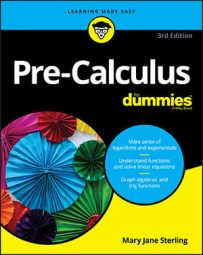If you need to find the limit of a function algebraically, you have four techniques to choose from: plugging in the x value, factoring, rationalizing the numerator, and finding the lowest common denominator.

The best place to start is the first technique. You can only use this technique if the function is continuous at the x value at which you are taking the limit. If the function is undefined at this x value, you must move on to the other techniques to simplify your function so that you can plug in the approached value for x.

## Find the limit by plugging in the x value

The first technique for algebraically solving for a limit is to plug the number that x is approaching into the function. If you get an undefined value (0 in the denominator), you must move on to another technique. But if your function is continuous at that x value, you will get a value, and you're done; you've found your limit! For example, with this method, you can find this limit: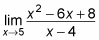The limit is 3, because f(5) = 3 and this function is continuous at x = 5.

## Find the limit by factoring

Factoring is the method to try when plugging in fails — especially when any part of the given function is a polynomial expression.

Say you're asked to find this limit: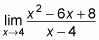You first try to plug 4 into the function, and you get 0 in the numerator and the denominator, which tells you to move on to the next technique. The quadratic expression in the numerator screams for you to try factoring it. Notice that the numerator of the previous function factors to (x – 4)(x – 2). The x – 4 cancels on the top and the bottom of the fraction. This step leaves you with f(x) = x – 2. You can plug 4 into this continuous function to get 2.

If you graph this function, it looks like the straight line f(x) = x – 2, but it has a hole when x = 4 because the original function is still undefined there (because it creates 0 in the denominator). The figure illustrates this.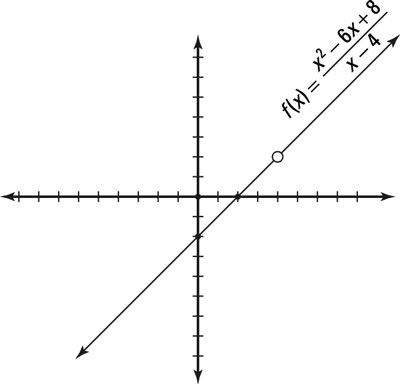If, after you've factored the top and bottom of the fraction, a term in the denominator didn't cancel and the value that you're looking for is undefined, the limit of the function at that value of x does not exist (which you can write as DNE).

For example, this function factors as shown: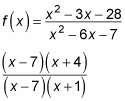The (x – 7) on the top and bottom cancel. So if you're asked to find the limit of the function as x approaches 7, you could plug 7 into the cancelled version and get 11/8. But if you're trying to find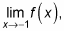the limit DNE, because you'd get 0 on the denominator. This function, therefore, has a limit anywhere except as x approaches –1.

## Find the limit by rationalizing the numerator

The third technique you need to know to find limits algebraically requires you to rationalize the numerator. Functions that require this method have a square root in the numerator and a polynomial expression in the denominator. For example, say you're asked to find the limit of this function as x approaches 13: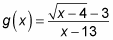Plugging in numbers fails when you get 0 in the denominator of the fraction. Factoring fails because the equation has no polynomial to factor. In this situation, if you multiply the numerator and denominator by the conjugate of the numerator, the term in the denominator that was a problem cancels out, and you'll be able to find the limit:

1. Multiply the top and bottom of the fraction by the conjugate.

The conjugate of the numerator is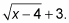Multiplying through, you get this setup: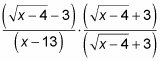Multiply the terms using the first, outer, inner, last (FOIL) technique in the numerator to get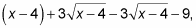which simplifies to x – 13 (the middle two terms cancel and you combine like terms from the FOIL).

2. Cancel factors.

Canceling gives you this expression: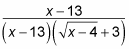The (x – 13) terms cancel, leaving you with this result: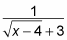3. Calculate the limits.

When you plug 13 into the function, you get 1/6, which is the limit.

## Find the limit by finding the lowest common denominator

When you're given a complex rational function, you use the fourth and final algebraic limit-finding technique. The technique of plugging fails, because you end up with a 0 in one of the denominators. The function isn't factorable, and you have no square roots to rationalize. Therefore, you know to move on to the last technique. With this method, you combine the functions by finding the least common denominator (LCD). The terms cancel, at which point you can find the limit.

For example, follow the steps to find the limit: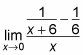1. Find the LCD of the fractions on the top.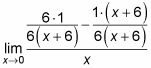2. Distribute the numerators on the top.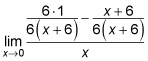3. Add or subtract the numerators and then cancel terms.

Subtracting the numerators gives you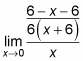which then simplifies to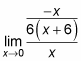4. Use the rules for fractions to simplify further.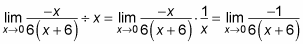5. Substitute the limit value into this function and simplify.

You want to find the limit as x approaches 0, so the limit here is –1/36.